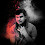### New Update

6/recent/ticker-posts

# CS8451 Design and Analysis of Algorithms Syllabus Notes Question Banks with answers

## CS8451 Design and Analysis of Algorithms Syllabus Notes Question Paper Question Banks with answers Anna University

#### Anna University Design and Analysis of Algorithms Syllabus Notes Question Bank Question Papers Regulation 2017

Anna University CS8451 Design and Analysis of Algorithms Notes are provided below. CS8451 Notes all 5 units notes are uploaded here. here CS8451 Design and Analysis of Algorithms notes download link is provided and students can download the CS8451 DAA Lecture Notes and can make use of it.

#### CS8451 Design and Analysis of Algorithms Syllabus Regulation 2017

UNIT I INTRODUCTION
Notion of an Algorithm – Fundamentals of Algorithmic Problem Solving – Important Problem Types – Fundamentals of the Analysis of Algorithmic Efficiency –Asymptotic Notations and their properties. Analysis Framework – Empirical analysis - Mathematical analysis for Recursive and Non-recursive algorithms - Visualization.
UNIT II BRUTE FORCE AND DIVIDE-AND-CONQUER
Brute Force – Computing an – String Matching - Closest-Pair and Convex-Hull Problems - Exhaustive Search - Travelling Salesman Problem - Knapsack Problem - Assignment problem. Divide and Conquer Methodology – Binary Search – Merge sort – Quick sort – Heap Sort - Multiplication of Large Integers – Closest-Pair and Convex - Hull Problems.
UNIT III DYNAMIC PROGRAMMING AND GREEDY TECHNIQUE
Dynamic programming – Principle of optimality - Coin changing problem, Computing a Binomial Coefficient – Floyd‘s algorithm – Multi stage graph - Optimal Binary Search Trees – Knapsack Problem and Memory functions.
Greedy Technique – Container loading problem - Prim‘s algorithm and Kruskal's Algorithm – 0/1 Knapsack problem, Optimal Merge pattern - Huffman Trees.
UNIT IV ITERATIVE IMPROVEMENT
The Simplex Method - The Maximum-Flow Problem – Maximum Matching in Bipartite Graphs, Stable marriage Problem.
UNIT V COPING WITH THE LIMITATIONS OF ALGORITHM POWER
Lower - Bound Arguments - P, NP NP- Complete and NP Hard Problems. Backtracking – n-Queen problem - Hamiltonian Circuit Problem – Subset Sum Problem. Branch and Bound – LIFO Search and FIFO search - Assignment problem – Knapsack Problem – Travelling Salesman Problem - Approximation Algorithms for NP-Hard Problems – Travelling Salesman problem – Knapsack problem..
Anna University internal Marks Out of 20 check here
Search by students
CS8451 Design and Analysis of Algorithms Regulation 2017 notes
Anna university UG Notes
Anna University Regulation 2017 Notes Syllabus
Regulation 2017 Syllabus Notes Anna University
CS8451 Design and Analysis of Algorithms Notes Syllabus
CS8451 Design and Analysis of Algorithms Notes
CS8451 Design and Analysis of Algorithms Syllabus
CS8451 Design and Analysis of Algorithms Question papers
CS8451 Design and Analysis of Algorithms Question Bank

1.2.3.4.5.Where is cs8451 important questions

6.Important question venum Tmw test ya

7.